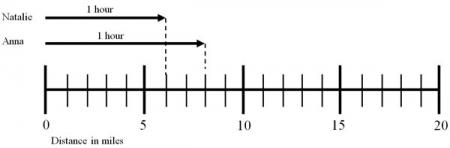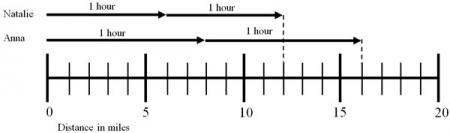# Let's Get Started

TEKS Standards and Student Objectives

A(2) Linear functions, equations, and inequalities. The student applies the mathematical process standards when using properties of linear functions to write and represent in multiple ways, with and without technology, linear equations, inequalities, and systems of equations. The student is expected to:

A(2)(C) write linear equations in two variables given a table of values, a graph, and a verbal description

Resource Objective(s)

Use a verbal description to create a table of values.

Determine the rate of change and y-intercept from a table of values.

Use a table of values to make predictions about unknown data.

Essential Questions

How can a table of values be generated from a verbal description?

How can the rate of change and y-intercept be determined from a table of values?

How can a table of values be used to make predictions about unknown data?

Vocabulary

When a table of data is given without a verbal description of the relationship, it is helpful to first determine the relationship between the data.

Crawfish

(Pounds)

Total Cost

(Dollars)

1 3
2 6
3 9
4 12
5 15

Looking at the table, a pattern is visible. Use the data in the table to fill in the blanks below.

Not all tables have a visible pattern—at times it is more difficult to see.

When this happens the rate of change can be calculated by dividing the change in the y-values (second value) by the change in the x-values (first value). The y-intercept can be found by calculating the initial value from the table. This relationship can be expressed as the equation.

 Length of Phone Call (Minutes) Total Cost  (Dollars) 1 $2.50 2$3.00 3 $3.50 4$4.00

# Prediction Activity

Often we can create a table of values from everyday situations. Use this interactive applet to see how the length of a table causes the number of chairs to change.

Choose the following settings in the applet:

• Mode: Exploration
• Table: 4 Chairs
• Arrangement: Straight line

Select the "Increase" and "Decrease" buttons, and observe the change in length of the table and number of chairs.

Use the interactive table activity to complete the data table and multiple choice questions below.

# Creating a Picture to Solve a Problem

One way to understand a problem is to draw a picture in order to create a table or a graph.

Example

Natalie and Anna are training for the Houston Marathon. Natalie can run six miles per hour, and Anna can run eight miles per hour. After two miles, how far have Natalie and Anna traveled?From the picture below, you can see how far each girl has run in one hour.Because the question is asking about their distance after two hours, it is necessary show multiple hours. See the picture below.